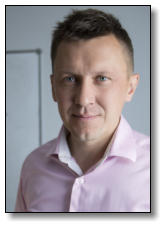Талалаев Дмитрий ВалерьевичSpecialization: Classical and quantum integrable systems. Exact solvable models of statistical mechanics. Topological invariants. Key words: Mathematical physics, algebraic geometry, quantum groups, invariants of knots and 2-knots. Teaching: Analytic geometry, linear algebra, differential geometry, training cours on computer geometry.Major research position at the Higher geometry and topology department of MSU(from 2009) Doctor habilitatus degree in physical-mathematical sciencesAdress: 119991 Moscow, MSU, faculty of mathematics and mechanics, higher geometry and topology department e-mail: dtalalaev@yandex.ru tel: (495) 939 3798